# Tidy data for the biology lab (Part 2)

This is the second of a two-part post. In Part 1, I introduced the concepts of tidy data and how to think about data formatting and transformation.

Part 2 (this section) is an in-depth tutorial using plate maps and readouts from a real 96-well plate experiment, including a notebook of working R code for processing and plotting the data.

# Tutorial: A 96-well plate assay

If you work in a lab you’ll eventually encounter a 96-well plate — this grid of 8 rows (labeled A through H) and 12 numeric columns is the workhorse of experimental biology. The “plate maps” of metadata (what you put in the wells) and the readouts of measurements per well (often by some type of “plate reader”) are often analyzed via 8x12 grids of cells in Excel mapping directly to the physical plate. Plate maps are often designed with some bespoke pattern of row, column and well text and colors in Excel. This format is intuitive for designing experiments, and makes a nice visual aid when pipetting, but quickly becomes an unwieldy nightmare later when it’s time to do the analysis. Let’s first tidy up the plate map into a flexible, analysis-ready metadata table.

## Platemap design

The first step is to think about the observational unit, in other words what are you perturbing and measuring? In a 96-well assay, you’ll be taking one measurement (or timecourse) per well, so let’s make the well the observational unit. If you’re taking multiple timepoints on each well, you’ll want a timepoint column, but if you don’t know the timepoints beforehand (e.g., if the plate reader assigns them), then we’ll deal with that later. Assign your well IDs as their own columns. It’s often handy to include a well ID like “H05” or “H5” but also separate row (“H”) and column (5) variables as well. Down the road this lets us explore batch effects like “striping” by plotting our data by plate rows and columns. If you have multiple plates, you should have a “plate” column as well.

Next think about the independent variables, or the metadata that went into the experimental design. What is the fundamental difference between samples? Are they different strains, sequence variants, or concentrations in a dilution? Create a table with the first “sample_id” column containing a unique identifier for each well, and then create columns for each sample attribute, such as treatments, sample type (positive control, negative control, test group), doses, timepoints. You’ll have less trouble later on if you just assign a sequential number, such as “sample_1”, “sample_2”, “sample_3”, but you can also embed metadata in the identifier, such as “strain3_3nM_24hr_rep3”.

Now for the most important part. Even though your “label” column `strain3_3nM_24hr_rep3` embeds the metadata, you should still also put “strain3” into a “strain” column, “3” into its own “dose (nM)” column, “24” into a “timepoint (hr)” column, and “3” into a “replicate” column. This allows the plotting tool to map these individual columns to facets, axes, color palettes, or other dimensions. Note that I put concentration units into the column labels so that the values can be numbers rather than text, enabling computations like curve fitting or log transformation. If you or a colleague will be writing code to analyze this data, it’s often better if you avoid punctuation (like parentheses) and use underscores, but these column names are ok for our purposes.

The nice thing about a metadata table, unlike an 8x12 grid, is that there is no limit to the number of annotations — you can add as many column attributes as you need — whereas the 8x12 plate map grid provides limited real estate to hold your metadata. Take advantage and give each attribute its own column, rather than embedding them into the sample label.

## Set up your data analysis workspace

Next we’ll demonstrate the R `tidyverse` package to show the power of tidy data commands and plotting.

To get started with R, there are only two steps: download and install the free RStudio Desktop application, then open the app and install the `tidyverse` package by running the command `install.packages('tidyverse')` in the console. Now you can run `library(tidyverse)` to load all the commands you’ll need.

RStudio has built-in notebooks, which are a really nice way to keep track of calculations and plots, for later publishing as html files. Almost all data scientists use computational notebooks in R (RMarkdown) or Python (Jupyter). Open a new notebook and check out the helpful template. This cheatsheet is a good starting point. Search the internet or RStudio docs for useful keyboard shortcuts for your OS, especially for creating and running code chunks.

Most likely, the data you get back from the plate reader will be untidy. Most importantly, you’ll want to format it to contain a well ID column that perfectly matches the well ID column in your metadata. With time-course data, this often requires a transpose operation, since well IDs are often used as columns. Your plate reader may also be able to export in tall table format, so use this setting if possible.

The example instrument data for this tutorial comes in untidy blocks:

You can copy and paste the block of timecourse data into a new, tidy table. I used the commands below from the R `readxl`, `tidyverse`, and `hms` packages to pull the data from Excel, transpose (gather) the well columns, and write to csv format. Although you’ll recognize some of the data verbs, I won’t be going over this script in detail (homework!).

`library(readxl)library(hms)library(tidyverse)df_480_p1 <- read_excel('data.xls', sheet=1, skip=30, n_max=16) %>%  gather(well, value, -Time, -temp) %>%  mutate(    plate = 1,     wavelength = emission_480,    timepoint = format(Time, '%H:%M:%S')) %>%  select(plate, well, timepoint, wavelength, value)# repeat for both plates and 530 wavelength block (not shown) df_480_p2 <- ...df_530_p1 <- ...df_530_p2 <- ...# Concatenate all plates/wavelengthsdf <- rbind(df_480_p2, df_480_p2, df_530_p1, df_530_p2) %>%  spread(wavelength, value)write_csv(df, 'readout.csv')`

Note that the plate number and well IDs are the only identifier, and you’ll need to use these to connect to the metadata from your plate map design. Keep this raw readout data pristine, in a separate table. We can merge in the metadata on demand, as we’ll show in the next section.

Having your data in tidy format continues to pay off when it’s time to create plots. I’ll use the popular `ggplot` package in R, but other tools such as Tableau, JMP, Spotfire, and the `seaborn` package in Python similarly create beautiful, interactive plots using tidy data.

Let’s plot the raw data now that we’ve tidied it up. I won’t go into the details of the `ggplot` code, since there are lots of tutorials online, but if you inspect the code carefully you’ll notice how we build up a visualization by mapping columns (time, emission_530, well) to aesthetics (x, y, line color, line group). You’ll also notice we use a`filter` data verb first before plotting. Plot layers are added with `+` . The last three layers deal with plot formatting, like adding a title, using a black-and-white theme, and rotating the `time` axis tick labels.

`plot_df <- filter(df, plate == 1, row == 'A')ggplot(plot_df, aes(x=time, y=emission_530, group=well, color=well)) +  geom_line() +  labs(title='Row A raw timecourse, 530nm') +  theme_bw() +  theme(axis.text.x = element_text(angle=90, hjust=0))`

Next, let’s zoom out and plot the raw timecourses for each well of the plate. We’ll facet by row and column to make a grid of plots for each well, and gather the two wavelength columns back into `wavelength` and `value` columns in order to simplify mapping the plot aesthetics — we can map `value` to the y-axis and `wavelength` to the color. This is the correct way to plot both on the y-axis at once.

`plate_1 <- filter(plate == 1)plot_df <- gather(plate_1, wavelength, value,                  emission_480, emission_530)ggplot(plot_df, aes(x=time, y=value,                     color=wavelength, group=wavelength)) +  facet_grid(row ~ col) +  geom_line() +  theme_bw() +  labs(title='Raw timecourse, plate 1') +  theme_bw() +  theme(axis.text.x = element_blank())`

There is much more we can do with the raw data, but we’ll move on. We have both metadata and raw readout data. How can we combine these, preprocess, perform calculations, and plot our data?

Now that we have one `csv` table for metadata and another for readout data, there are many ways to “join” or “merge” the two with one operation. Even if you have multiple readouts per well, a proper join simply copies the shared metadata to each row of the readout dataset. In R, the operation looks like this:

`# Load both tablesmeta = read_csv('meta.csv')readout = read_csv('readout.csv')# Join the datamerged = inner_join(meta, readout)`

The `inner_join` function automatically detects the shared column names and merges by those. If you want to merge on columns with different names, you can rename the columns beforehand with `rename` or tell `inner_join` what the shared keys are via additional arguments. The merged table now contains a row for each readout datapoint, with metadata columns pulled in and repeated for each row.

If you want to save the merged data to file, write to a new csv file, rather than overwriting the raw metadata or readout tables. Simply run `write_csv(merged, 'merged.csv')`

## Data processing

We have three calculations we want to make. First, we want to calculate the ratio of wavelengths for our BRET data. Then, we want to calculate the maximum value over time for each well. Finally, we’ll want to normalize by a plate-wide positive control value.

Creating the BRET column is easy in our tidy format, since it’s a columnwise operation on the readout data alone. Notice that within the `mutate` function, the column names are typed directly as variables. .

`bret <- mutate(readout, bret = emission_450/emission_530)`

Note that these `readout` column variables are not really available to you in your workspace (or “scope” in programming terms), but the function attaches them to the columns of `readout` on the fly. This “late binding” of function arguments is a big difference from languages such as Python, but one of many quirks of R that the `tidyverse` package leverages to create an elegant, interactive data language (though these same quirks make it frustrating as a general-purpose programming language).

Calculating maximum value over time, per well, is a grouped aggregation of our readout data.

`by_well <- group_by(bret, plate, well)max_per_well <- summarize(by_well, max_bret = max(bret))`

Now is the time to introduce chaining data verbs, which is a completely transformative mode of writing data code. The commands above are not too different to what the corresponding Python code might look like, and have the disadvantage of creating intermediate variables whose only job is to catch the output of one command and use it as the input to the next. Not only do you have to come up with names for these variables, but you have to type each one twice, which clutters your code and makes it harder to read. In the`tidyverse` package, all commands are designed to support “chaining” or “piping” from one command to the next, using the (admittedly weird) `%>%` operation.

This sounds mundane but take a look at the difference in readability for the exact same operations. First the old way, compiling the above commands:

`readout = read_csv('readout.csv')bret <- mutate(readout, bret = emission_450/emission_530)by_well <- group_by(bret, plate, well)max_per_well <- summarize(by_well, max_bret = max(bret))`

Now compare to the `tidyverse` chained style of operations. Notice that the first argument is dropped, so the function implicitly takes the output of the previous step.

`max_per_well <- read_csv('readout.csv') %>%    mutate(bret = emission_480/emission_530) %>%    group_by(plate, well) %>%    summarize(max_bret = max(bret))`

You can also pipe data directly into plots, which is a clean, efficient code pattern. You’ll see examples later.

Next, we want to normalize by the controls for each plate. Since we don’t know from the well ID which one is the control, this requires merging the metadata, followed by two additional calculations. First, we’ll perform a grouped aggregation on the per-well max BRET, to create a new summary table of average positive control values per plate. Then, we merge this summary table as a new column, so that each row has the corresponding value for its positive control. We can then normalize by taking the ratio of two columns. This may sound a bit complicated, and it would be in Excel, but it’s actually fairly easy using the data verbs you’ve already seen.

`avg_ctrl_by_plate <- max_per_well %>%    inner_join(meta) %>%    filter(sample == 'pos_ctrl') %>%    group_by(plate) %>%    summarize(avg_ctrl = mean(max_bret))normalized <- max_per_well %>%    inner_join(avg_ctrl_by_plate) %>%    mutate(norm_bret = max_bret / avg_ctrl)`

## Plot normalized data

Now we’ve summarized and normalized our data, it’s time to look at the results! Join the metadata back to the normalized per-well data to get sample information.

`ctrl_plot_data <- normalized %>%  inner_join(meta) %>%  filter(sample %in% c('Neg ctrl', 'Pos ctrl'))sample_plot_data <- normalized %>%  inner_join(meta) %>%  filter(!(sample %in% c('Neg ctrl', 'Pos ctrl')))ggplot(sample_plot_data, aes(x=log10(dose), y=norm_bret)) +   geom_boxplot(data=ctrl_plot_data, aes(fill=sample), width=0.5) +  geom_point(aes(color=sample)) +  geom_smooth(aes(color=sample),               method=drc::drm, method.args=list(fct=L.4()),               se = FALSE, alpha=0.5) +     labs(title='Dose response curves',        y='Normalized BRET', fill='control') +  theme_bw()`

# Next steps

If you’ve made it this far, then you’re starting to realize that there are huge advantages to the tidy approach to data analysis, and you’re itching to apply these techniques to your own data. Start by formatting your next dataset into tidy tables, and process these using Excel commands you’re familiar with. Learn more about pivot table functionality in Excel, as well as Table functionality.

When you’re ready to level up your programming skills, check out these nice cheatsheets for dplyr, ggplot2, and tidyr, summarizing the most common data munging, processing, and plotting operations. All of these commands are available when you import `tidyverse`. If you’d like to do more in-depth reading, start with Wickham’s R for Data Science, which he and co-author Garrett Grolemund have kindly open-sourced.

You may also want to learn Python and `pandas`, especially if you eventually want to learn programming in general or advanced models such as deep learning. However, R and `tidyverse `is more intuitive for beginners and is far better if you only want to do exploratory data analysis and plotting.

One major caveat: avoid learning “base” R (the core data functions that are built into the R language), which R tutorials on the web often teach. These commands have confusing syntax and don’t often work well with each other. Everything you need for normal data exploration, processing, and visualization is provided in the `tidyverse` package.

Code and data availability: I’d love to release a Github repo with the R notebook and data files from this project, but it would be a bit of work to clean it up. If I see enough interest in this post, either via >1000 claps or a handful of comments, then I’ll release the code and data.

--

--

--

## More from Jake Feala

Digitizing biology from hypothesis to experiment and back. Currently Head of Computational Biology at Generate Biomedicines. Twitter @FealaJake

Love podcasts or audiobooks? Learn on the go with our new app.

## Machine Learning Research Paper Summary: Unsupervised Video Summarization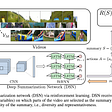## PCA : Choose your performers differently !!!!## Building a Recommendation System for Site Planning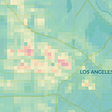## Popular Questions about the Data Engineering Career Path## How to Deliver on Your Data Strategy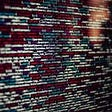## What it takes to be correlated## End to End Machine Learning Project on Used Car Prediction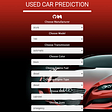## Backtesting statistics — SOG## Jake Feala

Digitizing biology from hypothesis to experiment and back. Currently Head of Computational Biology at Generate Biomedicines. Twitter @FealaJake

## Time Series Smoothing in R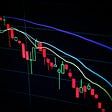## How to Make a Scatter Plot in R## Air Quality Dataset Plot with Plotly Using R Shiny Webapp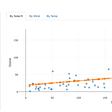## The Uses and Differences between ggplot2 and Plotly for Data Visualization with R